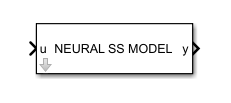# Neural State-Space Model

Simulate neural state-space model in Simulink

• Library:
• System Identification Toolbox / Models

•## Description

Simulates neural state-space (`idNeuralStateSpace`) models.

## Ports

### Input

expand all

Input of the neural state-space model, specified as a vector signal with as many elements as the number of inputs you specify when creating the corresponding `idNeuralStateSpace` object. If the `idNeuralStateSpace` object specified in the block dialog does not have inputs, this port does not appear.

Initial state of the neural state-space model, specified as a vector signal with as many elements as the number of states you specify when creating the corresponding `idNeuralStateSpace` object.

#### Dependencies

To enable this input port, select the initial state source parameter as `External`. Then, connect the port to an upstream signal supplying the value of the initial state.

### Output

expand all

Input of the neural state-space model, returned as a vector signal with as many elements as the number of outputs you specify when creating the corresponding `idNeuralStateSpace` object.

State of the neural state-space model, output as a vector signal with as many elements as the number of states nx that you specify when creating the corresponding `idNeuralStateSpace` object. These values are identical to the first nx values in the `y` output.

#### Dependencies

To enable this output port, select the Output state parameter.

State derivative or difference of the neural state-space model, output as a vector signal with as many elements as the number of states you specify when creating the corresponding `idNeuralStateSpace` object.

For continuous-time neural state space models, this value is the derivative of the state with respect to time. For discrete-time neural state space models, this value is the difference between the next state and the current one.

#### Dependencies

To enable this output port, select the Output state derivative/difference parameter.

## Parameters

expand all

Specify an `idNeuralStateSpace` object that defines a neural state-space system by entering the name of an `idNeuralStateSpace` object from the MATLAB® workspace.

Note

If you change the input/output configuration of `Model` in the workspace, click the button next to the Model edit field in the block dialog to refresh the Neural State-Space Model block.

#### Programmatic Use

 Block Parameter: `sys` Type: string, character vector Default: `""`

Specify whether to enter the initial condition directly on the dialog box or to receive the initial condition from an input port.

• If you set Initial state source to `Internal`, enter the initial condition in the edit field under Initial state.

• If you set Initial state source to `External`, the input port `x0` is added to the block. You must then connect an upstream signal supplying the value of the initial state to the `x0` input port.

#### Programmatic Use

 Block Parameter: `x0type` Type: character vector Values: `'Internal'` | `'External'` Default: `'Internal'`

Specify the initial state of the neural state-space object as a vector of nx entries, where nx is the number of states in `Model`.

Use this parameter make sure that the system states reflect the true plant environment at the start of your simulation, to the best of your knowledge.

#### Programmatic Use

 Block Parameter: `ic` Type: string, character vector Default: `"0"`

Select this parameter to add the x output port to the block. During simulation this port outputs the internal state of the `idNeuralStateSpace` object specified in `Model`.

#### Programmatic Use

 Block Parameter: `returnx` Type: string, character vector Values: `"off"`, `"on"` Default: `"off"`

Select this parameter to add the dx output port to the block. During simulation this port outputs the time derivative of the internal state of the `idNeuralStateSpace` object specified in `Model`.

#### Programmatic Use

 Block Parameter: `returndx` Type: string, character vector Values: `"off"`, `"on"` Default: `"off"`

## Version History

Introduced in R2022b